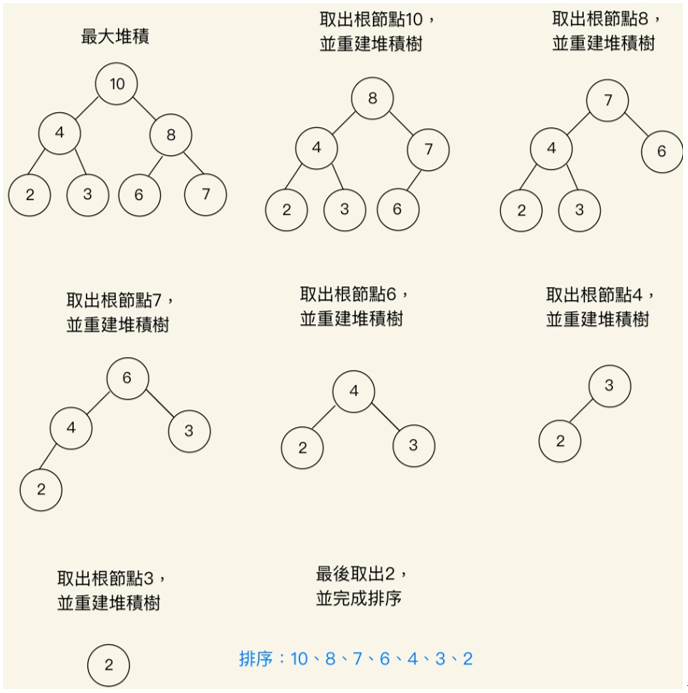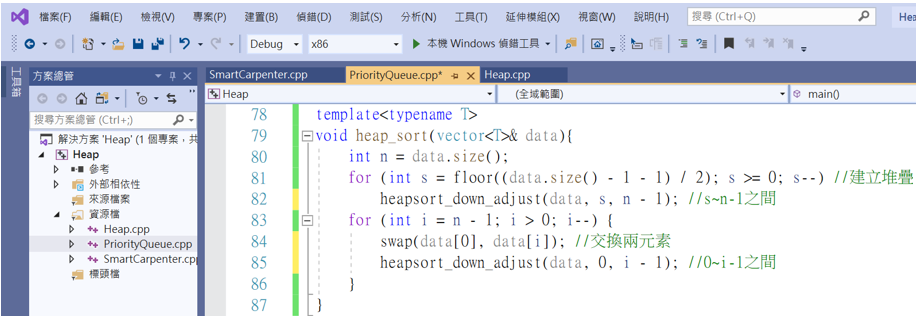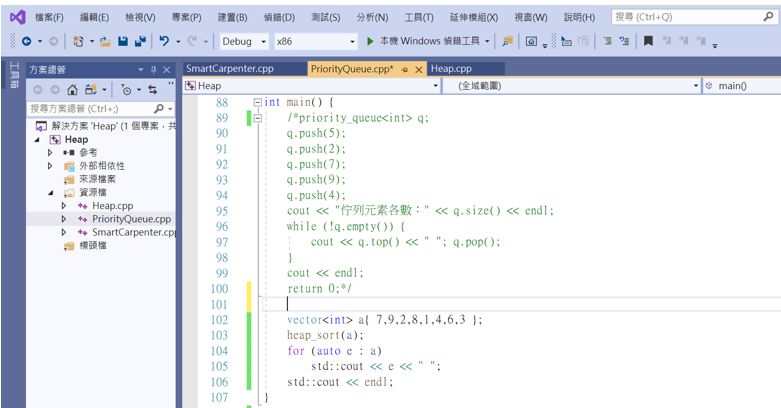#DAY 27
0
Software Development

## [Day27]程式菜鳥自學C++資料結構演算法 – 堆積排序法(Heap sort)

1. 從二元樹調整節點或建立成堆積。
2. 在輸出堆積的根節點後，將剩下的二元樹節點重建成堆積。
3. 重複操作直到所有節點輸出。``````template<typename T>
void heapsort_down_adjust(vector<T>& data, int s,int m) { //從下標為s的元素開始調整
T t = data[s];  //把s節點的資料放入t元素變量內，表示s最後的去處
for (int j = 2 * s + 1; j <= m;) { //先找到s的左子節點{j = 2 * s + 1}
if (j < m && data[j] < data[j + 1]) //j可想成節點的編號，data[j]則是節點內的資料
j++; //j指向較大的子節點
if (!(t < data[j])) break; //如果t(data[s])沒有小於data[j]，則跳出迴圈
data[s] = data[j]; //接著開始移動元素和指標
s = j; j = 2 * j;
}
data[s] = t;
}

template<typename T>
void heap_sort(vector<T>& data){
int n = data.size();
for (int s = floor((data.size() - 1 - 1) / 2); s >= 0; s--) //建立堆疊
heapsort_down_adjust(data, s, n - 1); //s~n-1之間
for (int i = n - 1; i > 0; i--) {
swap(data, data[i]); //交換兩元素
heapsort_down_adjust(data, 0, i - 1); //0~i-1之間
}
}
``````